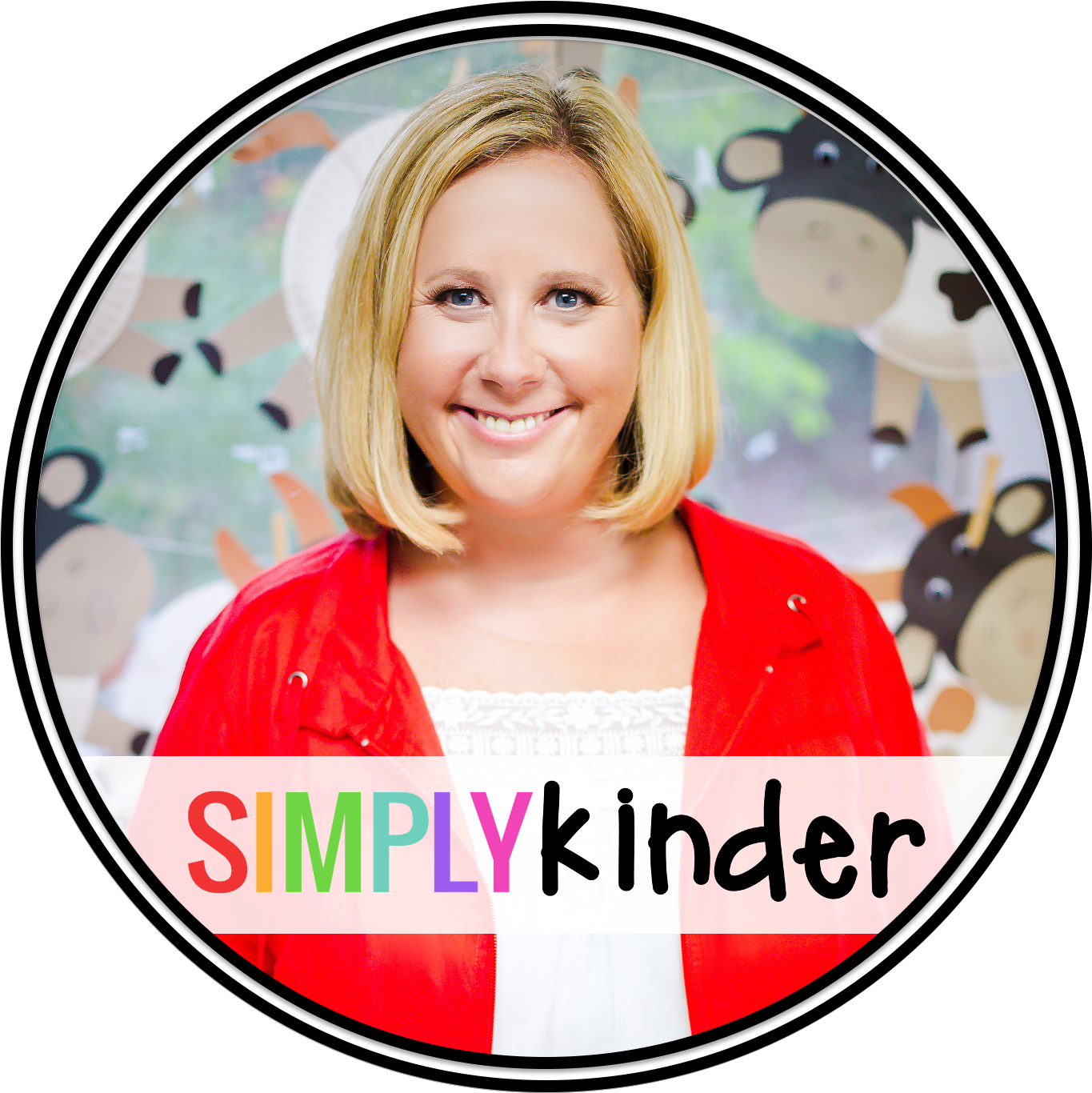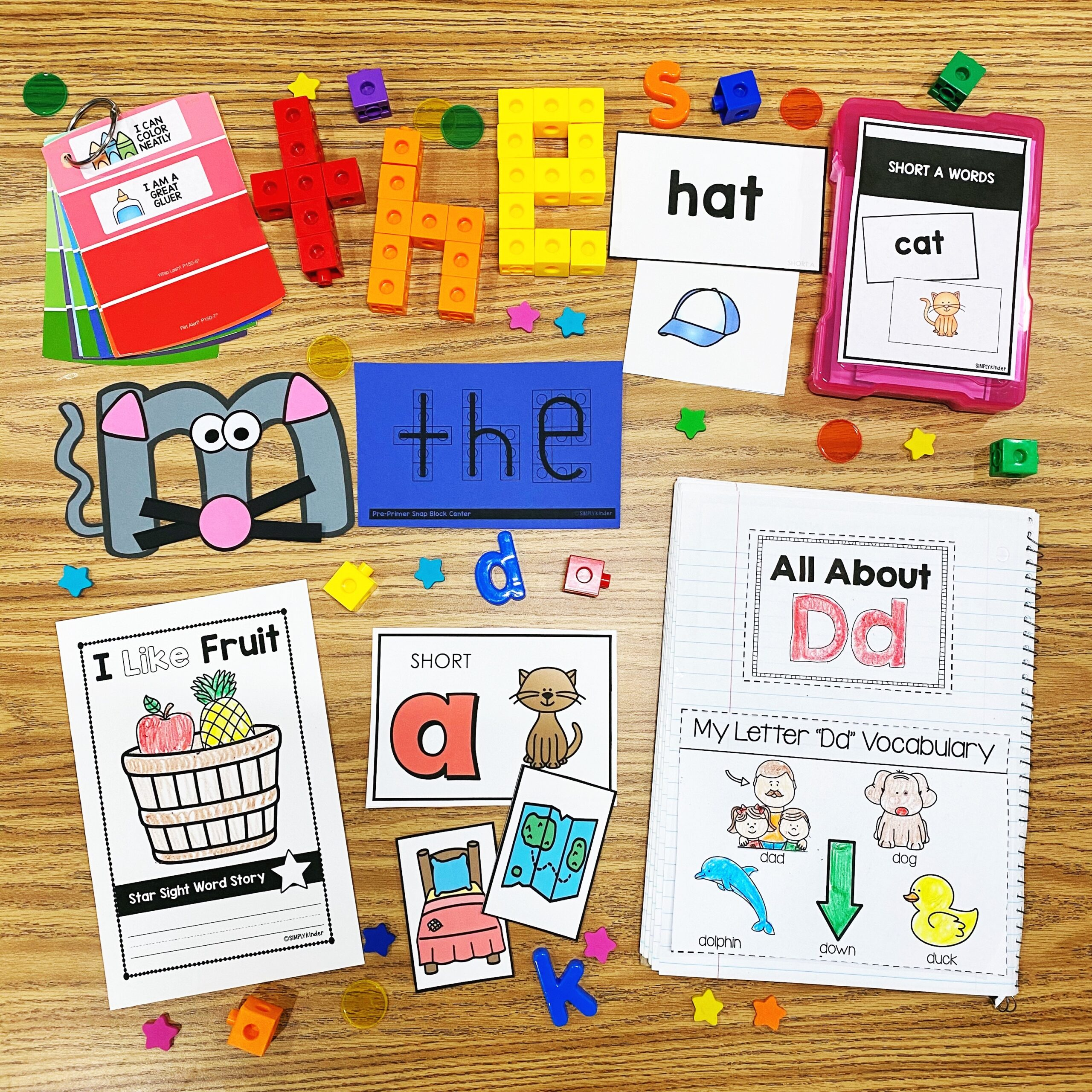Math Word Problems For Kinders to Tackle - Simply Kinder

# Math Word Problems For Kinders to Tackle

Check out these easy and fun FREE math word problems that are perfect for Kindergarten students. These interactive and engaging activities are common core aligned and will help students practice addition and subtraction skills. Keep reading to get tips and ideas on how to use these FREE math word problems in your classroom.

This resource works well with these Simply Kinder resources:

Teaching math word problems can be challenging for any teacher. Think about the multitude of skills students need to use to succeed. First they need to read and understand the text. Then apply the correct math concepts.

This is no easy task. But it is important for kids to learn. Think about it. We do not want students to simply memorize math facts. That only takes them so far.

Our goal is to help each student develop a conceptual understanding and then fluency. Exploring math through word problems helps build a deep understanding of the different operations.

## Benefits of Math Word Problems

Providing opportunities for students to engage in problem solving has multiple benefits. It helps

• Develops mathematical reasoning
• Provides opportunities to communicate mathematical thinking
• Gives ongoing practice with specific operations
• Keeps math interesting and relevant.

## Common Core Connections

As teachers it our responsibility to make sure all the common core standards are covered. So it is helpful to list those that are covered with each lesson.

1. Represent addition and subtraction with objects, fingers, mental images, drawings1, sounds (e.g., claps), acting out situations, verbal explanations, expressions, or equations.
2. Solve addition and subtraction word problems, and add and subtract within 10, e.g., by using objects or drawings to represent the problem.
3. Decompose numbers less than or equal to 10 into pairs in more than one way, e.g., by using objects or drawings, and record each decomposition by a drawing or equation

## What is word problem in mathematics?

A math word problem consists of a few sentences describing a scenario that needs to be solved using a mathematical calculation. This means that the important information is presented as text rather than numerals.

Today we are focusing on math word problems that involve addition and subtraction.

## The Types of Word Problems

There are 4 types of word problems students can solve. These are

1. Put together
3. Take from
4. Take apart

#### Put together

These problems involve two or more groups of objects. We put the groups together to find the total. These problems cover the basic concept of addition.

Suzie has 4 blocks and  Julia has  3  blocks. How many blocks do the girls have together?

These problems start with one group of objects and then add on another group. The result is a larger group. These problems help transition Kinders from counting every object to counting on. For example

There were three frogs in the pond, then 2 more frogs hopped into the pond. How many frogs do we have in the pond now?

Students could count every frog in the pond, but we want our students to understand  it is faster to start with 3 and count on to 5

#### Take From

In these problems we start with a group of objects. Then we take away some of the objects and are left with a smaller group than what we started with. This is the basic concept of subtraction.

Peter had 8 jellybeans in a bag. He ate 4 jellybeans from the bag. How many jellybeans are left in the bag?

#### Take apart

These problems start with a group know as the total. We break up the total into two or more smaller groups. Example

Kristen grew 8 flowers. 6 of her flowers were red and  the rest were yellow. How many flowers were yellow?

## How do you solve math problems for kids?

Our focus is helping our students to understand the problem that needs to be solved, rather than teaching the key words. Words like how many, altogether, or left can be helpful. But sometimes they can be misleading or missing.

## Use math word problems through the day

As you go through the school day, take the opportunity  to  use math problems. Take advantage of everyday examples to help build students’ understanding.

• We have 4 green cushions and 3 yellow cushions. How many cushions do we have altogether?
• I have 4 books. Who else needs to hand in their book? Now I have 5 more books. How many do I have altogether?
• We have 10 pencils in this box, and I will hand out 6 pencils. How many pencils will I have left in the box?
• I have 9 pencils in this box. 4 of them are green and the rest are yellow. How many are yellow?

## What are the 5 steps in solving word problems?

In Kindergarten we will encourage students to act out the scenarios, so they can see and experience the word problems. Start with classroom objects and then move onto worksheets with manipulatives to do this.

There are five main steps

2. Circle or underline all the numbers
3. Use manipulatives to act out the problem. Students can also draw it out.
4. Write the number sentence
5. Solve the number sentence

## Free Printable Worksheets

We’ve created some free printable worksheets to help students practice math word problems. There are four worksheets to cover each type of word problem.

The worksheets use multiple tools to help students solve the problem. They include paper manipulatives that must be cut out. Some of the worksheets use ten frames and others have number lines. Each worksheet encourages the students to write down the number sentence

#### Put together problems

These are the simplest math word problems and use paper manipulatives. Children will cut out the butterflies, glue them to the picture and count the total.

This worksheet uses a number line to help the children count on. They will also cut out the birds and place them on the branch.

#### Take From

The worksheet starts with the total number and takes objects away. Students will cut out 6 frogs, place the total down and then remove 2 from the pond.

#### Take apart

These are the hardest problems for Kinders to tackle. The worksheet provides a space to act out the problem. Children can cut out 8 flowers, color 6 of them red and the rest yellow. We’ve also included a ten sqaure. Use buttons or the flowers. Then fill out the blanks, create the number sentence and solve the equation.

## Some ideas for using these worksheets?

They would be great for morning work or homework. You could also use them as math warmups. Pair kids up to work on the problems together or send them home as homework.

## Looking for more math ideas?

Check out my math board on Pinterest for more activities and ideas,Welcome! It is our goal to help busy teachers just like you to be the best teachers they can be through our classroom ideas, resources, and community. ~ Jennifer

## Join the ListJoin thousands of other teachers and stay on top of all things kindergarten.  Receive a FREE 46 page resource to get started!

## NEW RESOURCES